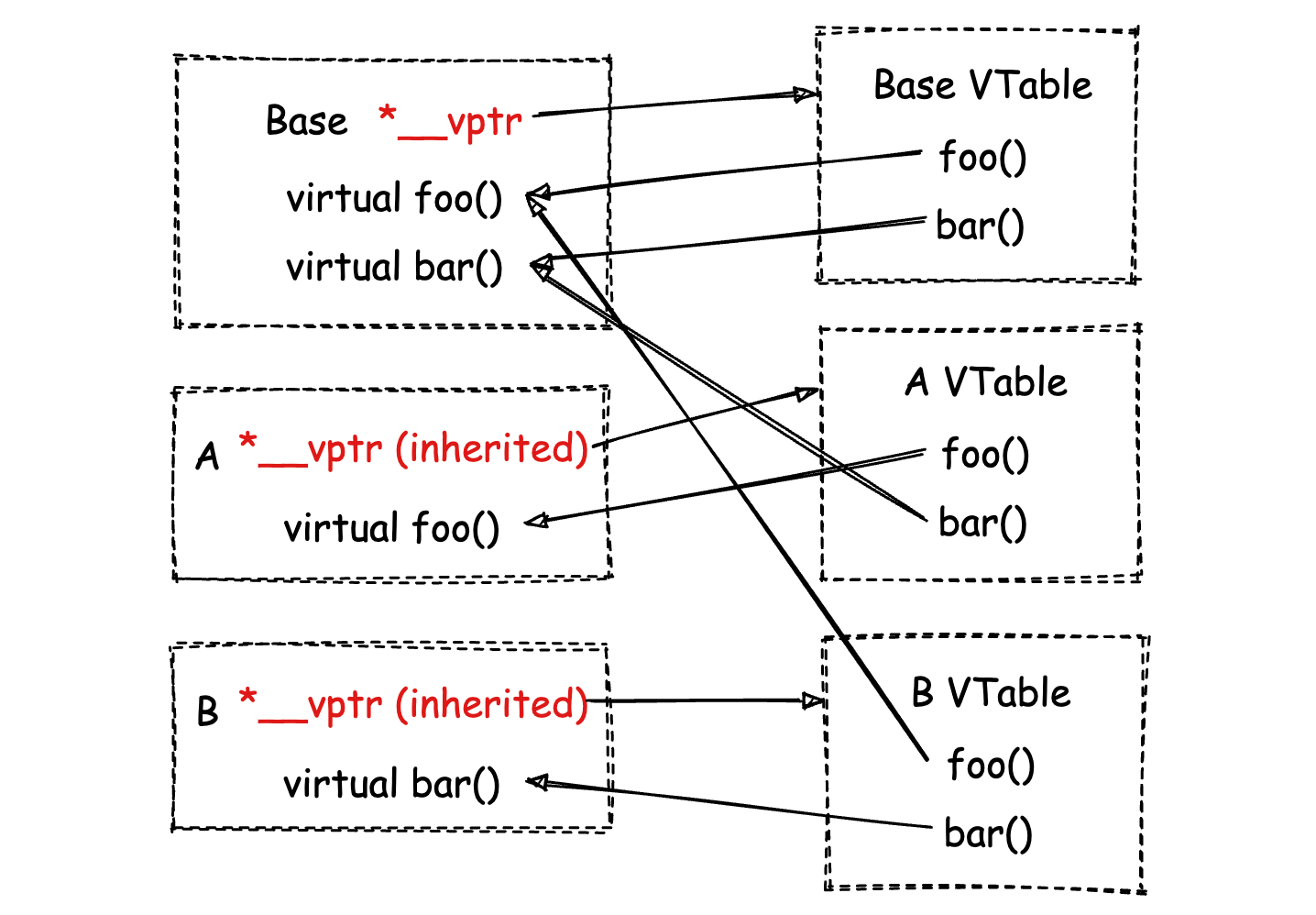X

# vtable 与 vptr

``````class Base {
public:
virtual void foo() {
std::cout << "Base::foo" << std::endl;
};
virtual void bar() {
std::cout << "Base::bar" << std::endl;
};
};
class A: public Base {
public:
void foo() {
std::cout << "A::foo" << std::endl;
};
};
class B: public Base {
public:
void bar() {
std::cout << "B::bar" << std::endl;
};
};
int main(int argc, char **argv) {
A a;
B b;
a.bar();  // "Base::bar".
b.bar();  // "B::bar".
a.foo();  // "A::foo".
b.foo();  // "Base::foo".
return 0;
}
``````